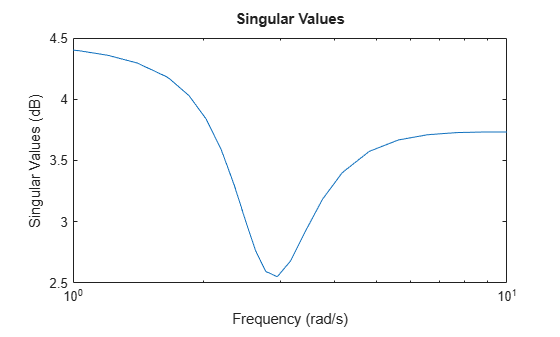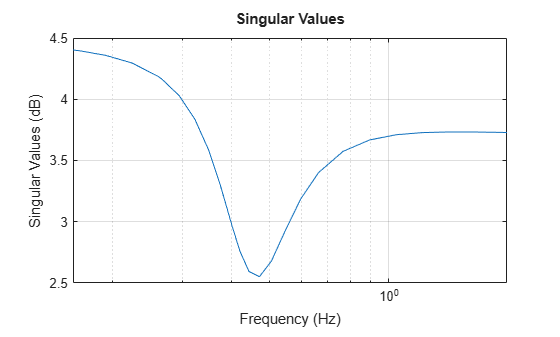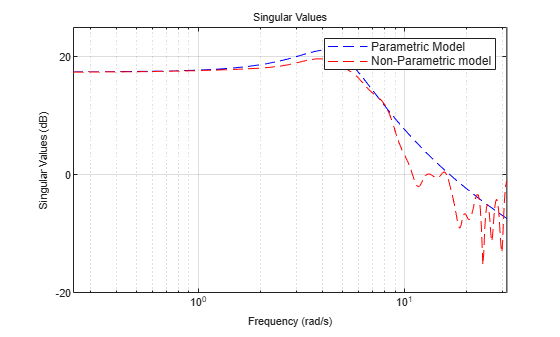# sigmaplot

Plot singular values of frequency response with additional plot customization options

## Syntax

``h = sigmaplot(sys)``
``h = sigmaplot(sys1,sys2,...,sysN)``
``h = sigmaplot(sys1,LineSpec1,...,sysN,LineSpecN)``
``h = sigmaplot(___,w)``
``h = sigmaplot(___,type)``
``h = sigmaplot(AX,___)``
``h = sigmaplot(___,plotoptions)``

## Description

`sigmaplot` lets you plot the singular values (SV) of frequency response of a dynamic system model with a broader range of plot customization options than `sigma`. You can use `sigmaplot` to obtain the plot handle and use it to customize the plot, such as modify the axes labels, limits and units. You can also use `sigmaplot` to draw an SV plot on an existing set of axes represented by an axes handle. To customize an existing SV plot using the plot handle:

1. Obtain the plot handle

2. Use `getoptions` to obtain the option set

3. Update the plot using `setoptions` to modify the required options

For more information, see Customizing Response Plots from the Command Line. To create SV plots with default options or to extract the frequency response data, use `sigma`.

example

````h = sigmaplot(sys)` plots the singular values (SV) of the frequency response of the dynamic system model `sys` and returns the plot handle `h` to the plot. You can use this handle `h` to customize the plot with the `getoptions` and `setoptions` commands.```

example

````h = sigmaplot(sys1,sys2,...,sysN)` plots the SV of multiple dynamic systems `sys1,sys2,…,sysN` on the same plot. All systems must have the same number of inputs and outputs to use this syntax.```

example

````h = sigmaplot(sys1,LineSpec1,...,sysN,LineSpecN)` sets the line style, marker type, and color for the SV plot of each system. All systems must have the same number of inputs and outputs to use this syntax.```

example

````h = sigmaplot(___,w)` plots singular values for frequencies specified by the frequencies in `w`.If `w` is a cell array of the form `{wmin,wmax}`, then `sigmaplot` plots the singular values at frequencies ranging between `wmin` and `wmax`.If `w` is a vector of frequencies, then `sigmaplot` plots the singular values at each specified frequency.You can use `w` with any of the input-argument combinations in previous syntaxes.See `logspace` to generate logarithmically spaced frequency vectors.```

example

````h = sigmaplot(___,type)` plots the modified singular value responses based on the `type` argument. Specify `type` as:`1` to plot the SV of the frequency response H-1, where H is the frequency response of `sys`.`2` to plot the SV of the frequency response I+H.`3` to plot the SV of the frequency response I+H-1.You can only use the `type` argument for square systems, that is, systems that have the same number of inputs and outputs.```
````h = sigmaplot(AX,___)` plots the singular values on the `Axes` object in the current figure with the handle `AX`.```

example

````h = sigmaplot(___,plotoptions)` plots the singular values with the options set specified in `plotoptions`. You can use these options to customize the SV plot appearance using the command line. Settings you specify in `plotoptions` overrides the preference settings in the MATLAB® session in which you run `sigmaplot`. Therefore, this syntax is useful when you want to write a script to generate multiple plots that look the same regardless of the local preferences.```

## Examples

collapse all

For this example, use the plot handle to change the frequency units to Hz and turn on the grid.

Generate a random state-space model with 5 states and create the sigma plot with plot handle `h`.

```rng("default") sys = rss(5); h = sigmaplot(sys);```Change the units to Hz and turn on the grid. To do so, edit properties of the plot handle, `h` using `setoptions`.

`setoptions(h,'FreqUnits','Hz','Grid','on');`The sigma plot automatically updates when you call `setoptions`.

Alternatively, you can also use the `sigmaoptions` command to specify the required plot options. First, create an options set based on the toolbox preferences.

`p = sigmaoptions('cstprefs');`

Change properties of the options set by setting the frequency units to Hz and enable the grid.

```p.FreqUnits = 'Hz'; p.Grid = 'on'; sigmaplot(sys,p);```You can use the same option set to create multiple sigma plots with the same customization. Depending on your own toolbox preferences, the plot you obtain might look different from this plot. Only the properties that you set explicitly, in this example `Grid` and `FreqUnits`, override the toolbox preferences.

For this example, create a sigma plot that uses 15-point red text for the title. This plot should look the same, regardless of the preferences of the MATLAB session in which it is generated.

First, create a default options set using `sigmaoptions`.

`plotoptions = sigmaoptions;`

Next, change the required properties of the options set `plotoptions`.

```plotoptions.Title.FontSize = 15; plotoptions.Title.Color = [1 0 0]; plotoptions.FreqUnits = 'Hz'; plotoptions.Grid = 'on';```

Now, create a sigma plot using the options set `plotoptions`.

`h = sigmaplot(tf(1,[1,1]),plotoptions);`Because `plotoptions` begins with a fixed set of options, the plot result is independent of the toolbox preferences of the MATLAB session.

For this example, create a sigma plot of the following continuous-time SISO dynamic system. Then, turn the grid on, rename the plot and change the frequency scale.

`$sys\left(s\right)=\frac{{s}^{2}+0.1s+7.5}{{s}^{4}+0.12{s}^{3}+9{s}^{2}}.$`

Create the transfer function `sys`.

`sys = tf([1 0.1 7.5],[1 0.12 9 0 0]);`

Next, create the options set using `sigmaoptions` and change the required plot properties.

```plotoptions = sigmaoptions; plotoptions.Grid = 'on'; plotoptions.FreqScale = 'linear'; plotoptions.Title.String = 'Singular Value Plot of Transfer Function';```

Now, create the sigma plot with the custom option set `plotoptions`.

`h = sigmaplot(sys,plotoptions);``sigmaplot` automatically selects the plot range based on the system dynamics.

For this example, consider a MIMO state-space model with 3 inputs, 3 outputs and 3 states. Create a sigma plot with linear frequency scale, frequency units in Hz and turn the grid on.

Create the MIMO state-space model `sys_mimo`.

```J = [8 -3 -3; -3 8 -3; -3 -3 8]; F = 0.2*eye(3); A = -J\F; B = inv(J); C = eye(3); D = 0; sys_mimo = ss(A,B,C,D); size(sys_mimo)```
```State-space model with 3 outputs, 3 inputs, and 3 states. ```

Create a sigma plot with plot handle `h` and use `getoptions` for a list of the options available.

`h = sigmaplot(sys_mimo);``p = getoptions(h)`
```p = FreqUnits: 'rad/s' FreqScale: 'log' MagUnits: 'dB' MagScale: 'linear' IOGrouping: 'none' InputLabels: [1x1 struct] OutputLabels: [1x1 struct] InputVisible: {0x1 cell} OutputVisible: {0x1 cell} Title: [1x1 struct] XLabel: [1x1 struct] YLabel: [1x1 struct] TickLabel: [1x1 struct] Grid: 'off' GridColor: [0.1500 0.1500 0.1500] XLim: {[1.0000e-03 1]} YLim: {[-25 15]} XLimMode: {'auto'} YLimMode: {'auto'} ```

Use `setoptions` to update the plot with the requires customization.

`setoptions(h,'FreqScale','linear','FreqUnits','Hz','Grid','on');`The sigma plot automatically updates when you call `setoptions`.

For this example, compare the SV for the frequencies of a parametric model, identified from input/output data, to a non-parametric model identified using the same data. Identify parametric and non-parametric models based on the data.

Load the data and create the parametric and non-parametric models using `tfest` and `spa`, respectively.

```load iddata2 z2; w = linspace(0,10*pi,128); sys_np = spa(z2,[],w); sys_p = tfest(z2,2);```

`spa` and `tfest` require System Identification Toolbox™ software. The model `sys_np` is a non-parametric identified model while, `sys_p` is a parametric identified model.

Create an options set to turn the grid on. Then, create a sigma plot that includes both systems using this options set.

```plotoptions = sigmaoptions; plotoptions.Grid = 'on'; h = sigmaplot(sys_p,'b--',sys_np,'r--',w,plotoptions); legend('Parametric Model','Non-Parametric model');```Consider the following two-input, two-output dynamic system.

`$H\left(s\right)=\left[\begin{array}{cc}0& \frac{3s}{{s}^{2}+s+10}\\ \frac{s+1}{s+5}& \frac{2}{s+6}\end{array}\right].$`

Plot the singular value responses of H(s) and I + H(s). Set appropriate titles using the plot option set.

```H = [0, tf([3 0],[1 1 10]) ; tf([1 1],[1 5]), tf(2,[1 6])]; opts1 = sigmaoptions; opts1.Grid = 'on'; opts1.Title.String = 'Singular Value Plot of H(s)'; h1 = sigmaplot(H,opts1);```Use input 2 to plot the modified SV of type, I + H(s).

```opts2 = sigmaoptions; opts2.Grid = 'on'; opts2.Title.String = 'Singular Value Plot of I+H(s)'; h2 = sigmaplot(H,[],2,opts2);```## Input Arguments

collapse all

Dynamic system, specified as a SISO or MIMO dynamic system model or array of dynamic system models. Dynamic systems that you can use include:

• Continuous-time or discrete-time numeric LTI models, such as `tf`, `zpk`, or `ss` models.

• Sparse state-space models, such as `sparss` or `mechss` models. Frequency grid `w` must be specified for sparse models.

• Generalized or uncertain LTI models such as `genss` or `uss` (Robust Control Toolbox) models. (Using uncertain models requires Robust Control Toolbox™ software.)

• For tunable control design blocks, the function evaluates the model at its current value to plot the SV.

• For uncertain control design blocks, the function plots the SV at the nominal value and random samples of the model.

• Frequency-response data models such as `frd` models. For such models, the function plots the SV at frequencies defined in the model.

• Identified LTI models, such as `idtf` (System Identification Toolbox), `idss` (System Identification Toolbox), or `idproc` (System Identification Toolbox) models. For such models, the function can also plot confidence intervals and return standard deviations of the frequency response. (Using identified models requires System Identification Toolbox™ software.)

Line style, marker, and color, specified as a character vector or string containing symbols. The symbols can appear in any order. You do not need to specify all three characteristics (line style, marker, and color). For example, if you omit the line style and specify the marker, then the plot shows only the marker and no line.

Example: `'--or'` is a red dashed line with circle markers

Line StyleDescription
`-`Solid line
`--`Dashed line
`:`Dotted line
`-.`Dash-dot line
MarkerDescription
`'o'`Circle
`'+'`Plus sign
`'*'`Asterisk
`'.'`Point
`'x'`Cross
`'_'`Horizontal line
`'|'`Vertical line
`'s'`Square
`'d'`Diamond
`'^'`Upward-pointing triangle
`'v'`Downward-pointing triangle
`'>'`Right-pointing triangle
`'<'`Left-pointing triangle
`'p'`Pentagram
`'h'`Hexagram
ColorDescription

`y`

yellow

`m`

magenta

`c`

cyan

`r`

red

`g`

green

`b`

blue

`w`

white

`k`

black

Frequencies at which to compute and plot SV of the frequency response, specified as the cell array `{wmin,wmax}` or as a vector of frequency values.

• If `w` is a cell array of the form `{wmin,wmax}`, then the function plots the SV at frequencies ranging between `wmin` and `wmax`.

• If `w` is a vector of frequencies, then the function plots the SV at each specified frequency. For example, use `logspace` to generate a row vector with logarithmically spaced frequency values.

Specify frequencies in units of rad/`TimeUnit`, where `TimeUnit` is the `TimeUnit` property of the model.

Option to plot modified singular values, specified as one of the following options:

• `1` to plot the SV of the frequency response H-1, where H is the frequency response of `sys`.

• `2` to plot the SV of the frequency response I+H.

• `3` to plot the SV of the frequency response I+H-1.

You can only use the `type` argument for square systems, that is, systems that have the same number of inputs and outputs.

Target axes, specified as an `Axes` object. If you do not specify the axes and if the current axes are Cartesian axes, then `sigmaplot` plots on the current axes.

Sigma plot options set, specified as a `SigmaPlotOptions` object. You can use this option set to customize the SV plot appearance. Use `sigmaoptions` to create the option set. Settings you specify in `plotoptions` overrides the preference settings in the MATLAB session in which you run `sigmaplot`. Therefore, `plotoptions` is useful when you want to write a script to generate multiple plots that look the same regardless of the local preferences.

For the list of available options, see `sigmaoptions`.

## Output Arguments

collapse all

Plot handle, returned as a `handle` object. Use the handle `h` to get and set the properties of the SV plot using `getoptions` and `setoptions`. For the list of available options, see the Properties and Values Reference section in Customizing Response Plots from the Command Line.

## Version History

Introduced before R2006a